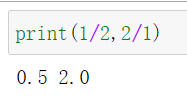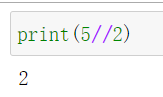Python 数据类型：数值和算术操作符

一，算术操作符

• +  ：加
• -  ：减
• *  ：乘
• **  ：幂乘，例如，a**b，是指a的b次方
• /  ：除，在Python3.0中，是真除法，无论任何类型都会保持小数部分
• //  ：Floor除法，省略掉结果的小数部分
• %  ：求模，求余数

二，数值类型概述

Python的数字类型包括：整数、浮点数、固定精度的小数、分数和复数：

• 整数（int）：在Python3.0中支持无穷精度的整数，可以通过内置函数int(n)来创建整数，整数是精确的，
• 浮点数（float）：类似于C语言中的双精度，可以通过内置函数float(n)来创建浮点数，浮点数可能存在误差，浮点数缺乏精确性
• 精确小数（decimal）：小数具有固定的位数和小数点
• 分数（fraction）：实现了有理数，它明确的保留了分字和分母，从而避免了浮点数字的不确定性。
• 复数：虚部以j或J结尾，可以通过内置函数complex（real,image)来创建复数
• 二进制，八进制和十六进制：可以通过内置函数bin(n)，oct(n)，hex(n)来分别创建二进制数，八进制数和十六进制数

1，整数（int）

Python支持十进制，八进制，二进制和十六进制的整数。

2，浮点数（float）

3，精确小数（decimal）

>>> 0.1+0.1+0.1-0.3
5.551115123125783e-17

>>> from decimal import Decimal
>>> Decimal('0.1') + Decimal('0.1') - Decimal('0.2')
Decimal('0.0')

decimal.getcontext().prec = 4

float和decimal是不同类型的小数，不能直接乘积和相除，

unsupported operand type(s) for *: 'float' and 'decimal.Decimal'

4，分数fraction）

>>> from fractions import Fraction
>>> x=Fraction(1,2)

三，整数类型

• 二进制（binary）：以0b或0B开头，后面接0-1的数字
• 八进制（octal）：以0o或0O开头，后面接0-7的数字，
• 十六进制（hex ）：以0x或0X开头，后面接十六进制的数字0-9和A-F，字符编写成大写或小写都行

• 常量0o10 是指十进制的8，使用oct(8)来表示
• 常量0b10是指十进制的2，使用bin(2)来表示
• 常量ox10是指十进制的16，使用hex(16)来表示

1，真除法（/）

x/y，真除法，在任何时候，数值的真除法会保留小数部分。2，Floor除法 （//）

Floor除法（操作符是 // ）是指：不考虑操作对象的类型，总是把结果向小舍入到最近的整数，例如，小于2.5最小的整数是2，小于-2.5最小的整数是-3>>> import math
>>> math.floor(2.5)
2
>>> math.floor(-2.5)
-3

/ 表示传统除法：如果两个操作数都是整数类型，执行截断的整数除法，返回的结果是整数；如果任意一个操作数是浮点数，则返回小数。

// 表示Floor除法：总是截断余数，当两个操作数都是整数时，结果是整数（n）；如果有任何一个操作数是浮点类型，则返回一个浮点数（n.0）。

/ 表示真除法：不管操作数的类型，都返回包含小数的结果；

// 表示Floor除法：对于整数执行截断除法，对于浮点数执行浮点除法。

>>> 5//2.0
2.0
>>> 5//-2.0
-3.0

四：精确小数

decimal表示精确小数，小数的位数（即小数的精度）是确定的，常用于需要精确小数的计算中。

from decimal import Decimal

decimal模块中的工具可以用来设置decimal的精度，

decimal.getcontext().prec = 4

import decimal
from decimal import Decimal

decimal.getcontext().prec=4

d=Decimal(1.0)/3
print(d)

#0.3333

五，内置的数学工具

• pow(a,b)，求a的b次幂
• round(seed)：求随机数
• math.sqrt(x)，求x的平方根
• math.floor(x)：向下取最小的整数
• math.trunc(x)：截断小数点，保留整数

posted @ 2018-12-11 13:08  悦光阴  阅读(1750)  评论(0编辑  收藏  举报